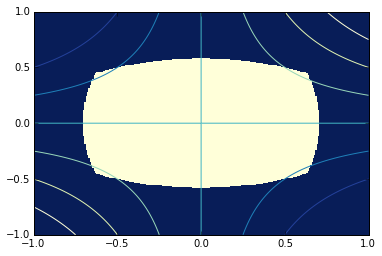## Exercise 1

1. Plot the functions $x^3$ and $\sin(x)$ on the interval $[0,1]$. You should see that they intersect somewhere between $0.9$ and $1.0$. Try computing the intersection point using either fsolve or newton in scipy.optimize.

2. Try setup a multivariable system and using fsolve to compute an intersection of the two ellipses

$$4x^2 + y^2 = 1 \\ x^2 + 9y^2 = 1$$

## Exercise 2

1. Using the minimum function, compute the minimum of the scalar function in two ways: with and without providing the Jacobian. $$f(x, y) = (x-1)^2 + (y-2)^2 - 3x + 2y + 1$$

2. How many steps should Newton's method for optimization take on a quadratic form?

## Exercise 3

Try using scipy.linprog to solve the linear program:

Maximize $x + y$ subject to \begin{align} 50x + 24y &\le 2400 \\ 30x + 33y &\le 2100 \end{align}

## Exercise 4

Try to maximize the function $xy$ constrained to the region:

$$2x^2 + y^2 = 1 \\ x^2 + 3y^2 = 1$$

To get a sense of what we're looking at, it may be useful to make a crude 2D plot indicating the constraint region and contours.

In :
X, Y = np.meshgrid(np.linspace(-1, 1, 256), np.linspace(-1, 1, 256))

plt.figure()
plt.pcolormesh(X, Y, (2*X**2 + Y**2 <= 1) & (X**2 + 3*Y**2 <= 1))
plt.contour(X, Y, X*Y)
plt.show()## Exercise 5

In this problem, we'll try doing a model fit with nonlinear least squares. In particular, we'll use curve_fit in scipy.optimize which provides a convinient interface for model fitting type problems.

In order to use it, we must provide a model function. In this problem, we'll be interested in fitting a "sum of Gaussians". More specifically, we know that our curve is of the form:

$$f(x, a_1, c_1, a_2, c_2) = a_1 e^{-(x-c_1)^2} + a_2 e^{-(x-c_2)^2}$$

We've collected some data as $(x,y)$-pairs stored in "gaussians.txt" and we believe this model should be an appropriate fit. Try reading the data, writing this function $f$ and passing that information to curve_fit(f, x, y) to estimate the parameters $a_1, c_1, a_2, c_2$.